• PCA the most popular for DR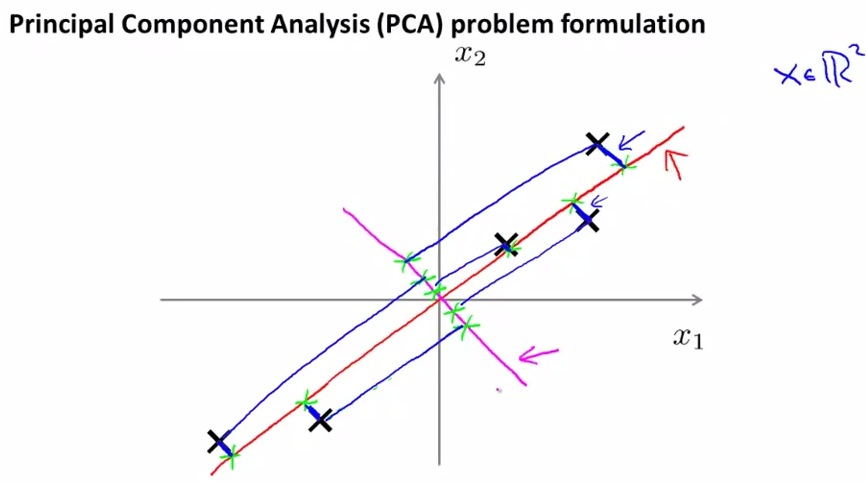• This is the graph  that we know how to make the projection line.
• PCA try to visualize where input should project to line, hows the length of error of each element, and minimialized it
• Second line in magenta is much worse
• PCA should more prefer red over magenta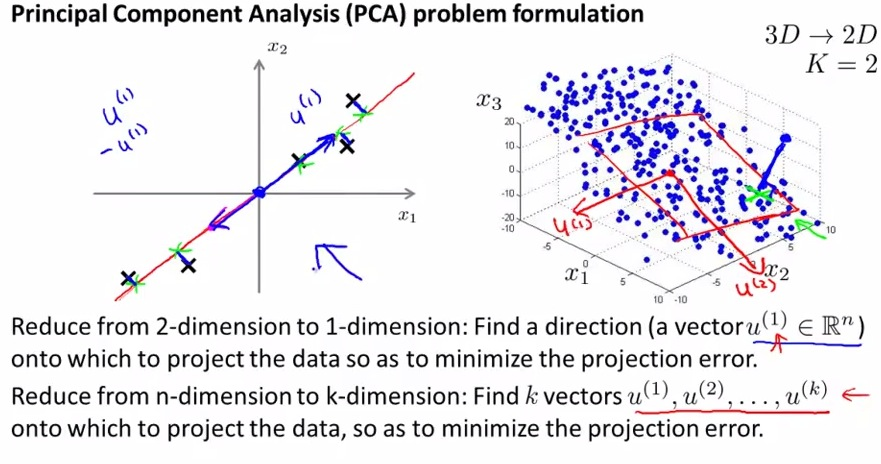• find the direction of ui (either positive or negative) and see if the projection line has the same direction, and try to minimalized the error
• Find pair of vector that project 2D-graph(plane)   of data to visualize it
• Try to minimalized the error between 3d position of the input and the 2D plane projection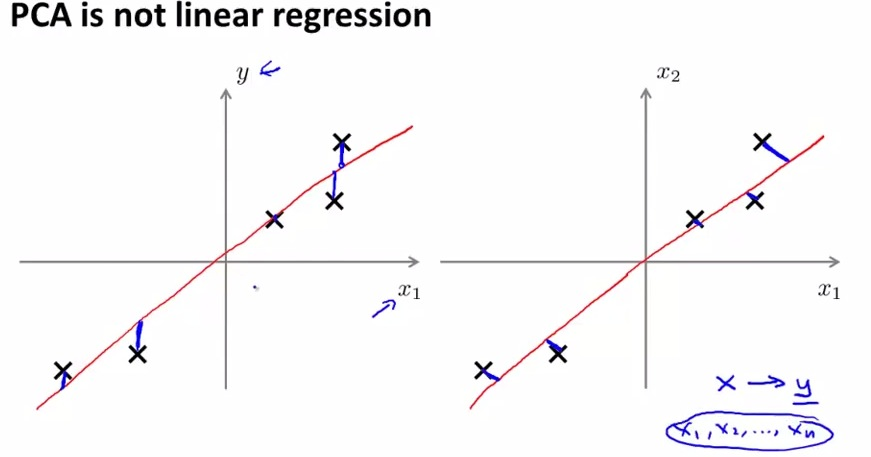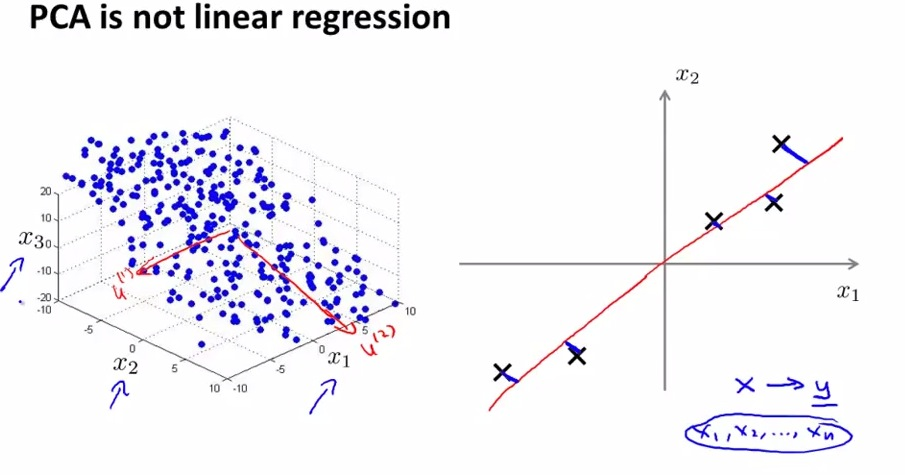• Similar to linear regression? No.
• Linear regression try to minimalized the vector between element and the projection line
• PCA try to minimalized the MAGNITUDE between element and projection line
• As we can see, the PCA can be diagonal (any straight line that closer to projectioin line, with angle
• aparameters considered evenly
• Linear regression trying to "predict y" based on x
• There's no special variable (ie LR has "y"). All parameters treated equally

• So PCA is trying to find a generalized 2D plane that project all the data. And also find the error and minimalize it from each point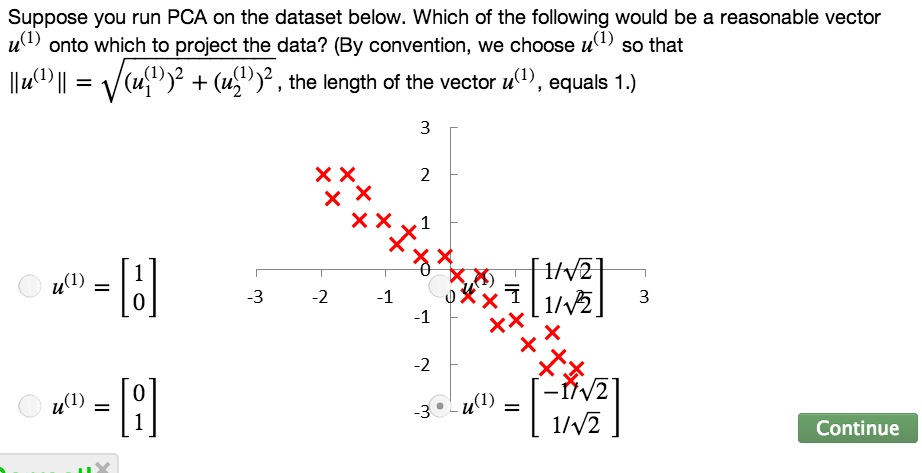• Next, what it's actually does